# The deepest point in the ocean is 11 km below sea level, deeper than Mt. Everest is tall

The deepest point in the ocean is 11 km below sea level, deeper than Mt. Everest is tall.

What is the pressure in atmospheres at this depth?

Concepts and reason
The concepts required to solve the given questions is the pressure in the atmosphere at the depth.

Initially, calculate the depth form km to m. Later, write an expression for the pressure at the depth. Finally, calculate the pressure in the atmosphere at this depth.

Fundamentals
The expression for the atmosphere at some depth is as follows:

Here, is the initial atmospheric pressure, D is the density of water, g is the acceleration due to gravity, and h is the deepest point in the ocean.

The depth in km is equal to,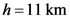Convert it into m as follows:Explanation:
The depth of the ocean is converted from km to m on multiplying by a factor of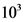.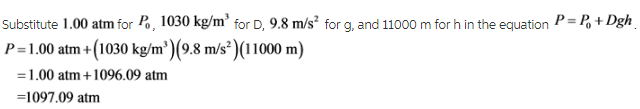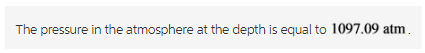Explanation:

The density of the water is equal to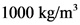. But we have to calculate the depth of the point in the ocean. The ocean contains salt water so, we have to use density of the salt water.